# KSEEB Solutions for Class 8 Maths Chapter 8 Linear Equations in One Variable Ex 8.1

In this chapter, we provide KSEEB SSLC Class 8 Maths Chapter 8 Linear Equations in One Variable Ex 8.1 for English medium students, Which will very helpful for every student in their exams. Students can download the latest KSEEB SSLC Class 8 Maths Chapter 8 Linear Equations in One Variable Ex 8.1 pdf, free KSEEB SSLC Class 8 Maths Chapter 8 Linear Equations in One Variable Ex 8.1 pdf download. Now you will get step by step solution to each question.

### Karnataka State Syllabus Class 8 Maths Chapter 8 Linear Equations in One Variable Ex 8.1

1. Solve the following:

Question i.
x + 3 = 11
x + 3 = 11
x = 11 – 3
x = 8

Question ii.
y – 9 = 21
y – 9 = 21
y = 21 + 9
y = 30

Question iii.
10 = z + 3
10 = z + 3 10-3 =z
7 = z or z = 7

Question iv.
311+x=911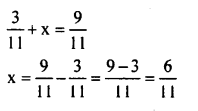Question v.
10x = 30
10x = 30
x = 3010
x = 3

Question vi.
s7=4
s7=4
S = 4 × 7
S = 28

Question vii.
3×6=10
3×6=10
3x = 10 × 6
3x = 60
x = 603
x = 20

Question viii.
1.6=x1.5
1.6=x1.5
1.6 × 1.5 = x
2.40 = x
x = 2.4

Question ix.
8x – 8 = 48
8x – 8 = 48
8x = 48 + 8
8x = 56
x = 568
x = 7

Question x.
x3+1=715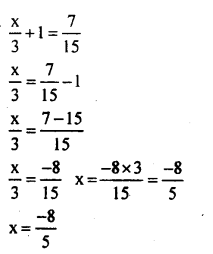Question xi.
x5=12
x5=12
x = 12 × 5
x = 60

Question xii.
3×5=15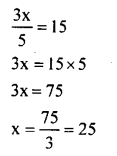Question xiii.
3(x + 6) = 24
3(x + 6) = 24
3x+ 18 = 24
3x = 24 – 18
3x = 6
x = 63 = 2

Question xiv.
x4−8=1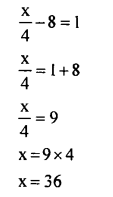Question xv.
3(x+2) – 2(x—1) = 7
3(x + 2) – 2(x – 1) = 7
3x + 6 – 2x + 2 = 7
x + 8 = 7 .
x = 7 – 8
x = -1

2. Solve the equations :

Question i.
5x = 3x + 24
5x = 3x + 24
2x = 24
x = 242
x = 12

Question ii.
8t + 5 = 2t – 31
8t + 5 = 2t – 31
6t = -36
t = −366
t = -6

Question iii.
7x -10 = 4x + 11
7x – 10 = 4x + 11
7x – 4x = 11 + 10
3x = 21
x = 213
x = 7

Question iv.
4z + 3 = 6 + 2z
4z + 3 = 6 + 2z
4z – 2z = 6 – 3
2z = 3

Question v.
2x – 1 = 14 – x
2x – 1 = 14 – x
2x + x = 14 + 1
3x = 15
x = 153
x = 5

Question vi.
6x + 1 = 3(x -1) + 7
6x + 1 = 3(x —1) + 7
6x + l =3x-3 + 7
6x + l = 3x + 4
6x – 3x = 4 – 1
3x = 3
x = 33
x = 1

Question vii.
2×5−32=x2+1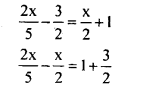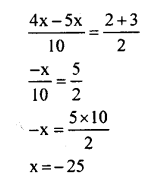Question viii.
x−35−2=2×5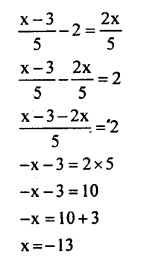Question ix.
3(x + 1) = 12 + 4(x – 1)
3(x + 1)= 12 + 4(x – 1)
3x + 3 = 12 + 4x – 4
3x + 3 = 4x + 8
3x – 4x = 8 – 3
-x = 5
x = -5

Question x.
2x – 5 = 3(x – 5)
2x – 5 = 3(x – 5)
2x – 5 = 3x – 15
2x – 3x = -15 + 5
-x = -10
x= 10

Question xi.
6(1 – 4x) + 7(2 + 5x) = 53
6( 1 – 4x) + 7(2 + 5x) = 53
6 – 24x+ 14 + 35x = 53
35x – 24x + 6 + 14 = 53
11x+ 20 = 53
11x = 53 – 20
x = 3311
x = 3

Question xii.
3(x + 6) + 2(x + 3) = 64
3(x + 6) + 2(x + 3) = 64
3x + 18 + 2x + 6 = 64
3x + 2x + 18 + 6 = 64
5x + 24 = 64
5x = 64 – 24
5x = 40
x = 405
∴ x = 8

Question xiii.
2m3+8=m2−1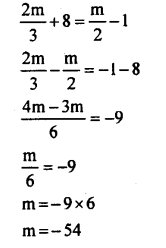Question xiv.
34(x−1)=(x−3)
34(x−1)=(x−3)
3(x – 1) = 4(x – 3)
3x – 3 = 4x – 12
3x – 4x = -12 + 3
-x = -9
x = 9

All Chapter KSEEB Solutions For Class 8 maths

—————————————————————————–

All Subject KSEEB Solutions For Class 8

*************************************************

I think you got complete solutions for this chapter. If You have any queries regarding this chapter, please comment on the below section our subject teacher will answer you. We tried our best to give complete solutions so you got good marks in your exam.

If these solutions have helped you, you can also share kseebsolutionsfor.com to your friends.

Best of Luck!!